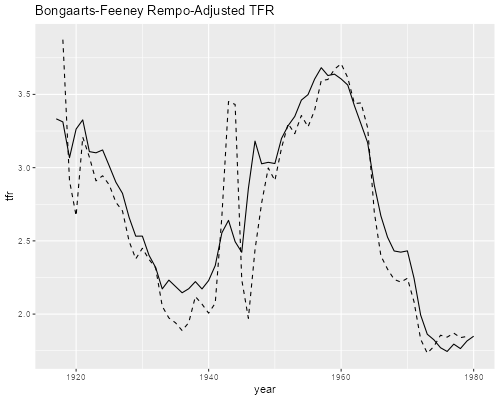Germán Rodríguez
Demographic Methods Princeton University## Fertility in the U.S. 1917-1980

We will use the Heuser cohort fertility tables available in the OPR data archive to have a look at fertility in the U.S. between 1917 and 1980. For a much more extensive analysis see the paper by Schoen (2004) “Timing Effects and the Interpretation of Period Fertility”, Demography 41(4):801-819, available in JSTOR. Also, the human fertility database has data for 1933-2013 and can be used to extend this series to the more recent past.

I did some preliminary work to put the archive data in a more usable form. The file `heuser.dat` in the datasets section is a 36 by 64 matrix of single-year age-period rates for ages 14 to 49 and years 1917 to 1980. This is easy to read into our software.

```. infile y1917-y1980 using ///
>   https://grodri.github.io/datasets/heuser.dat, clear

. gen age = 13.5 + _n  // ages 14.5 to 49.5
```
```> library(dplyr)
> heuser_wide <- mutate(heuser_wide, age = 13.5 + row_number())
> # will convert immediately to long format
> library(tidyr)
> heuser <- gather(heuser_wide, key="year", value="asfr", -age)
> heuser <- mutate(heuser,  year = 1916 + as.numeric(substr(year,2,3)))
```

In the sections below we will be looking at period and cohort fertility, but it is important to remember that we are dealing with an age-period-cohort surface. In class we will use dynamic 3-D graphs to explore the entire surface.

### Period Fertility

You may want to plot the age-specific fertility rates for selected years, for example 1935, 1945 and 1955

```. line y1935  y1945  y1955 age,   lp(solid longdash dash) ///
>   title(U.S. Age-Specific Period Fertility) ///
>   legend(order(1 "1935" 2 "1945" 3 "1955") ring(0) col(1) pos(1))

. graph export uspasfr.png, width(500) replace
file uspasfr.png saved as PNG format
```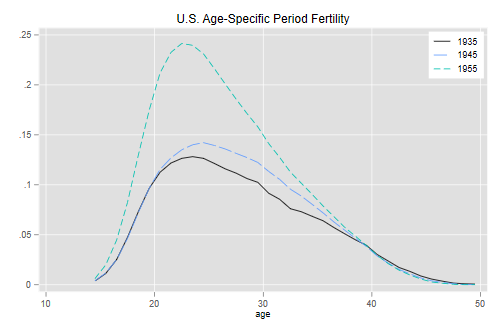```> library(ggplot2)
> selected <- filter(heuser, year==1935 | year == 1945 | year == 1955) |>
+   mutate(year = as.factor(year))
> ggplot(selected, aes(age, asfr, color=year)) + geom_line() +
+   ggtitle("U.S. Age-Specific Period Fertility")
> ggsave("uspasfrr.png", width = 500/72, height = 400/72, dpi = 72)
```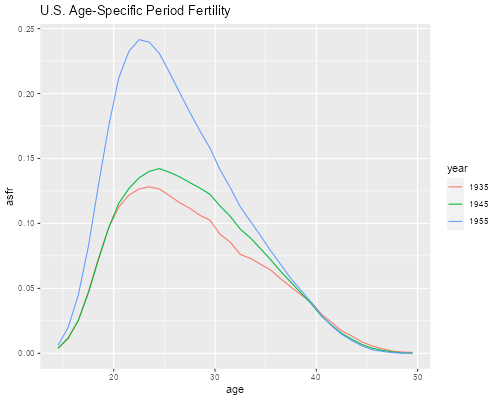The TFRs shows an increase over these years, from 2.2 to 3.5 children per woman.

```. tabstat y1935 y1945 y1955, stat(sum)

Stats │     y1935     y1945     y1955
─────────┼──────────────────────────────
Sum │    2.1887    2.4218    3.4983
─────────┴──────────────────────────────
```
```> group_by(selected, year) |> summarize(tfr = sum(asfr))
# A tibble: 3 × 2
year    tfr
<fct> <dbl>
1 1935   2.19
2 1945   2.42
3 1955   3.50
```

Let us compute the TFR and the mean age of childbearing for all years (named `tfr` and `mac`).

```. set obs 64 // make room  for 64 years
Number of observations (_N) was 36, now 64.

. mata:
───────────────────────────────────────────────── mata (type end to exit) ──────
:   rates = st_data(1::36, 1..64)

:   ones = J(36,1,1)

:   tfr = ones'rates

:   ages = st_data(1::36,"age")

:   mac = ages'rates :/ tfr

: end
────────────────────────────────────────────────────────────────────────────────

. gen period = 1916 + _n
```
```> periods <- group_by(heuser, year) |>
+   summarize(tfr = sum(asfr), mac = weighted.mean(age, asfr))
```

We can now plot the TFR and the mean age of childbearing, which we will do in separate panels

```. line tfr period, name(tfr,replace) title(Total Fertility Rate)

. line mac period, name(mac,replace) title(Mean Age of Childbearing)

. graph combine tfr mac,  xsize(6) ysize(3) ///
>   title("U.S. Period Fertility") name(periods)

. graph export ustfrmac.png, width(750) replace
file ustfrmac.png saved as PNG format
```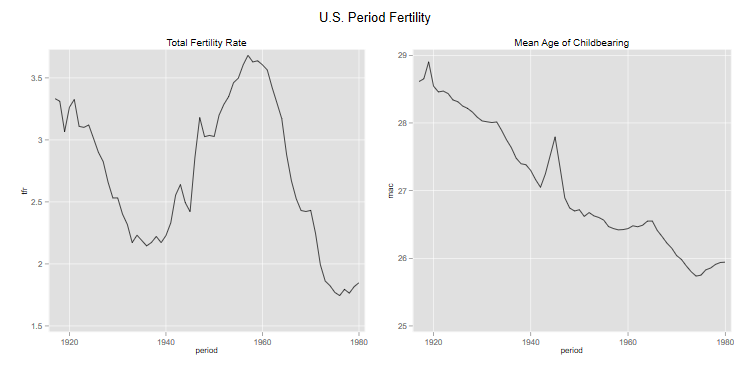```> library(gridExtra)
> p1 <- ggplot(periods, aes(year, tfr)) + geom_line() + ggtitle("Period TFR")
> p2 <- ggplot(periods, aes(year, mac)) + geom_line() + ggtitle("Period MAC")
> g <- arrangeGrob(p1, p2, ncol = 2)
> ggsave("ustfrmacr.png", plot = g, width = 10, height = 5, dpi = 72)
```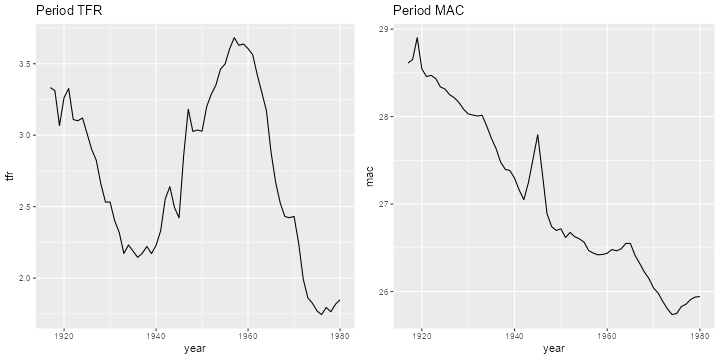We see that the decline in fertility betwen 1920 and 1940 was followed by the famous baby boom, while period mean age of childbearing declined steadily except for a blip after the war.

### Cohort Fertility

The data contain the complete fertility experience of 29 cohorts, starting with the cohort of 1903, aged 14 at the start of the series in 1917, and ending with the cohort of 1930, aged 49 at the end of the series in 1980.

To compute cohort summaries we need to extract the appropriate diagonal entries from the age-period array of rates and store them as new variables called c1903 to c1917

```. mata:
───────────────────────────────────────────────── mata (type end to exit) ──────
:   ageperiod = st_data(1::36,1..64)

:   nages = rows(ageperiod)

:   ncohorts = cols(ageperiod) - nages + 1

:   cohort = J(nages,1,0)

:   for (j = 1; j <= ncohorts; j++) {
>     for (i=1; i <= nages; i++) {
>       cohort[i] = ageperiod[i,i+j-1]
>     }
>     name = "c" + strofreal(1902+j)
>   }

: end
────────────────────────────────────────────────────────────────────────────────
```
```> heuser <- mutate(heuser, cohort = year - age + 0.5)
```

Here is a plot of cohort age-specific fertility for the cohorts born in 1910, 1920 and 1930, which were at their peak fertility ages in the period plotted earlier

```. line c1910 c1920 c1930 age, lp(solid longdash dash) ///
>   title(U.S. Age-Specific Cohort Fertility) ///
>   legend(order(1 "1910" 2 "1920" 3 "1930") ring(0) col(1) pos(1))

. graph export uscasfr.png, width(500) replace
file uscasfr.png saved as PNG format
```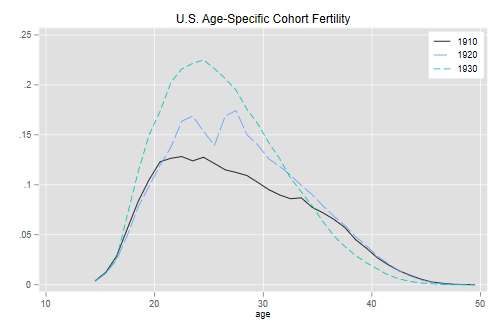```> selected <- filter(heuser, cohort == 1910 | cohort == 1920 | cohort == 1930) |>
+ mutate(cohort = as.factor(cohort))
> ggplot(selected, aes(age, asfr, color=cohort)) + geom_line() +
+   ggtitle("U.S. Age-Specific Cohort Fertility")
> ggsave("uscasfrr.png", width = 500/72, height = 400/72, dpi = 72)
```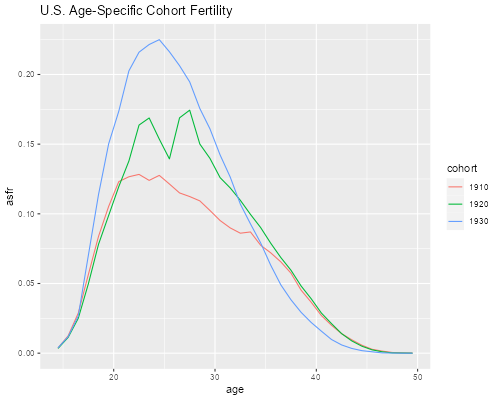We will use the same sort of calculation we did for periods to compute cohort total fertility rates and cohort mean ages of childbearing for the cohorts with complete experience, 1903 to 1931. (One could package these operations as separate functions for routine use.)

```. mata:
───────────────────────────────────────────────── mata (type end to exit) ──────
:   cols = st_varindex("c1903")..st_varindex("c1931")

:   rates = st_data(1::36,cols)

:   ones = J(36,1,1)

:   ctfr = ones'rates

:   ages = st_data(1::36,"age")

:   cmac = ages'rates :/ ctfr

: end
────────────────────────────────────────────────────────────────────────────────

. gen cohort = 1902 + _n in 1/29
(35 missing values generated)
```
```> cohorts <- filter(heuser, cohort >= 1903, cohort <= 1931) |>
+   group_by(cohort) |>
+   summarize(tfr = sum(asfr), mac = weighted.mean(age, asfr))
```

We now have the data needed for our plot

```. line ctfr cohort, name(ctfr,replace) title(Total Fertility Rate)

. line cmac cohort, name(cmac,replace) title(Mean Age of Childbearing)

. graph combine ctfr cmac, xsize(6) ysize(3) ///
>   title("U.S. Cohort Fertility")

. graph export uscohorts.png, width(750) replace
file uscohorts.png saved as PNG format
```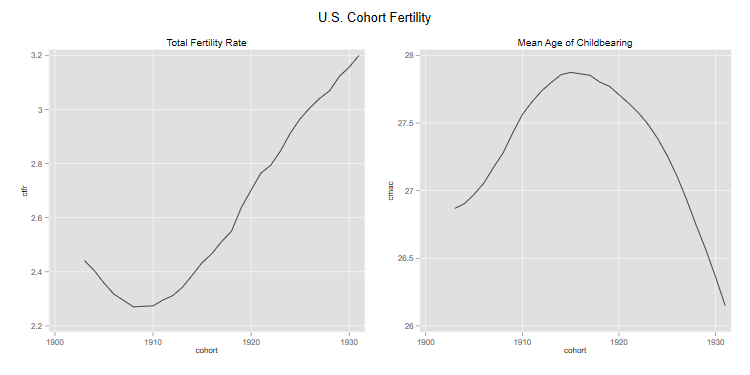```> c1 <- ggplot(cohorts, aes(cohort, tfr)) + geom_line() + ggtitle("Cohort TFR")
> c2 <- ggplot(cohorts, aes(cohort, mac)) + geom_line() + ggtitle("Cohort MAC")
> g <- arrangeGrob(c1, c2, ncol = 2)
> ggsave("uscohortsr.png", plot = g, width = 10, height = 5, dpi = 72)
```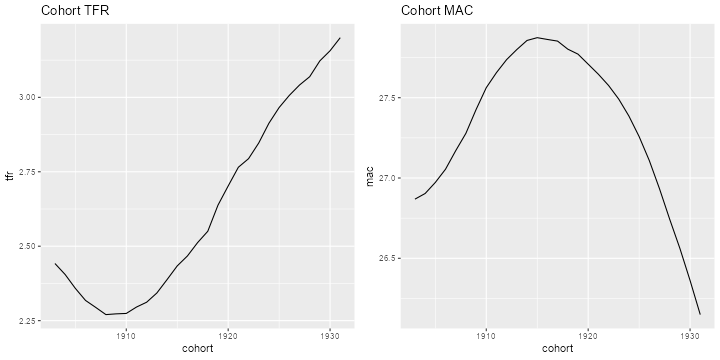As you can see, the story from the cohort perspective looks a bit different, particularly in terms of mean age of childbearing, which increased and then declined steadily.

### Ryder’s Translation

How can we reconcile these results? Let us start by plotting the period and cohort TFRs on the same graph. To do this we follow Ryder in dating a cohort’s fertility using its mean age of childbearing. The cohort of 1903 had a TFR of 2.44 with a mean age of childbearing of 26.9 so we plot this TFR in 1929.9.

```. gen cc = 1902 + _n + cmac // cc for cohort centroid
(35 missing values generated)

. twoway (line tfr period ) (line ctfr cc, lp(dash)), ///
>   legend (order(1 "period" 2 "cohort") ring(0) pos(7)) ///
>   title("U.S. Cohort and Period Total Fertility Rates")

. graph export uspctfr.png, width(500) replace
file uspctfr.png saved as PNG format
```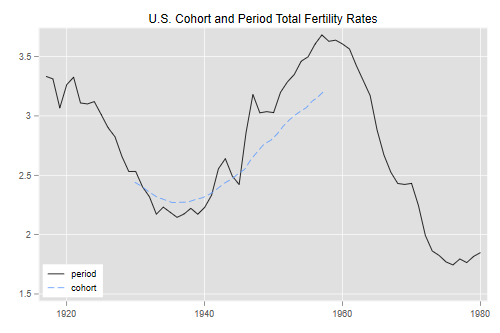```> cohorts <- mutate(cohorts, year = cohort + mac)
> ggplot(periods,aes(year,tfr)) + geom_line() +
+   geom_line(aes(year,tfr), data=cohorts, linetype=2) +
+   ggtitle("U.S. Cohort and Period Total Fertility Rates")
> ggsave("uspctfrr.png", width = 500/72, height = 400/72, dpi = 72)
```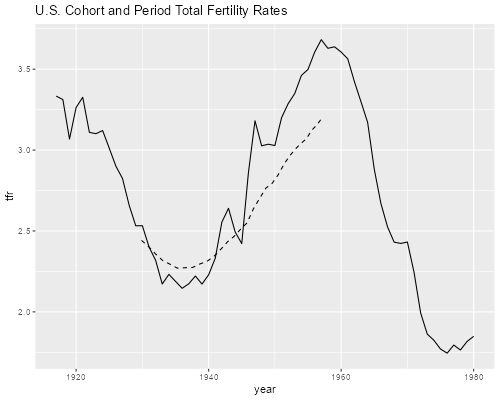We see that period fertility was lower than cohort fertility when the cohorts were delaying childbearing, and higher than cohort fertility when the cohorts moved childbearing to younger ages, leading to the babyboom. This, of course, is exactly what one would expect from first principles.

Ryder shows that, to a first order of approximation, the ratio of period to cohort TFR’s is given by one minus the first derivative of cohort mean age of childbearing, so

CTFR = TFR/(1 - rc)

with all cohort quantities evaluated for the cohort reaching its mean of childbearing in the period of interest. We can easily see how well this works, bearing in mind that a first order approximation will be exact only if the rates change linearly over time.

We need a way to compute numerical derivatives. B-F recommend estimating a derivative at time t by averaging changes between t-1 and t and between t and t+1, which works out to be the same as half the change between t-1 and t+1

```. mata:
───────────────────────────────────────────────── mata (type end to exit) ──────
:   real colvector nder(real colvector v) {
>     n = length(v)
>     d = (v[3::n] - v[1::(n-2)] )/2
>     return( . \ d \ . )
>   }

: end
────────────────────────────────────────────────────────────────────────────────
```
```> nder <- function(v) {
+   n <- length(v)
+   c(NA, (v[3:n] - v[1:(n-2)])/2, NA)
+ }
```

We now use this function to compute the derivative of the cohort mean ages of childbearing

```. mata:
───────────────────────────────────────────────── mata (type end to exit) ──────
:   mac = st_data(1::29,"cmac")

:   d = nder(mac)

: end
────────────────────────────────────────────────────────────────────────────────
```
```> cohorts <- mutate(cohorts, rc = nder(mac))
```

We now take the cohort rates, multiply them by 1-rc to obtain a period translation, and plot them at the cohort’s mean age of childbearing. The result should be an approximation to the period TFR:

```. gen ctr = ctfr*(1 - rc)   // ctr for cohort translation
(37 missing values generated)

. line tfr period || line ctr cc, lp(dash) || line ctfr cc, lp(longdash) ///
>   title("Ryder's Demographic Translation") ///
>   subtitle("U.S. Fertility 1917-1980") ///
>   legend(order(1 "period" 2 "cohort" 3 "translation") row(1) ring(0) pos(7))

. graph export usryder.png, width(500) replace
file usryder.png saved as PNG format
```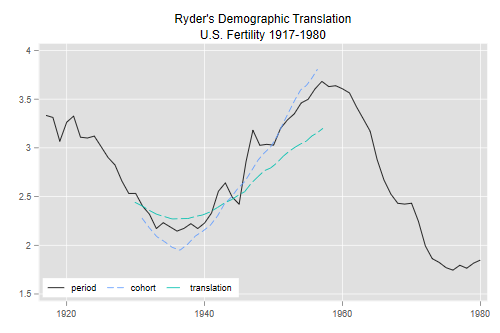```> cohorts <- mutate(cohorts, ctr = tfr * (1 - rc))
> ggplot(periods,aes(year,tfr)) + geom_line() +
+   geom_line(aes(year,tfr), data=cohorts, linetype=2)  +
+   geom_line(aes(year,ctr), data=cohorts, linetype=3) +
+   ggtitle("Ryder's Demograhic Translation - U.S. 1917-1980")
> ggsave("usryderr.png", width = 500/72, height = 400/72, dpi = 72)
```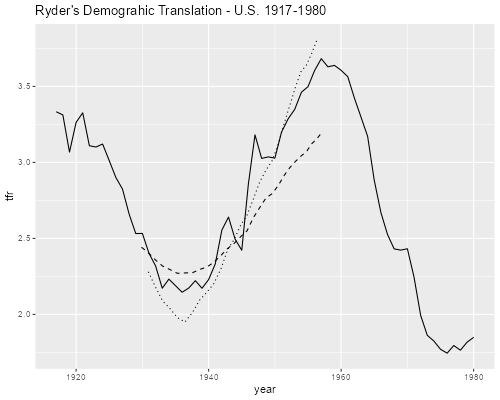The translation is smoother than the observed period TFR, but seems to track it reasonably well, usually identifying correctly times where the period TFR is above or below the completed family size of the relevant cohort.

Bongaarts and Feeney proposed adjusting the period TFR by dividing it by one minus the first derivative of period mean age of childbearing, so

TFR* = TFR/(1-rp).

Let us calculate this measure to see what it does. Note that B-F prefer calculating the adjustment separately by birth order using rates that divide births of a given order by all women. I will simply use all births and refer you to Schoen’s paper for the results using B-F’s preferred method. The paper by Keilman discusses the use of hazard rates.

We start by computing the derivative of period mean age of childbearing using our handy numerical derivative function, and then use this to compute the adjusted TFR

```. mata:
───────────────────────────────────────────────── mata (type end to exit) ──────
:   mac = st_data(1::64,"mac")

:   rp = nder(mac)

: end
────────────────────────────────────────────────────────────────────────────────

. gen tfrs = tfr/(1-rp)   // tfrs for TFR*
(2 missing values generated)

. twoway (line tfr period) (line tfrs period, lp(dash)),  ///
>   subtitle("U.S. Fertility 1917-1980") ///
>   legend(order(1 "TFR" 2 "TFR-BF") ring(0) pos(7))

. graph export ustfrbf.png, width(500) replace
file ustfrbf.png saved as PNG format
```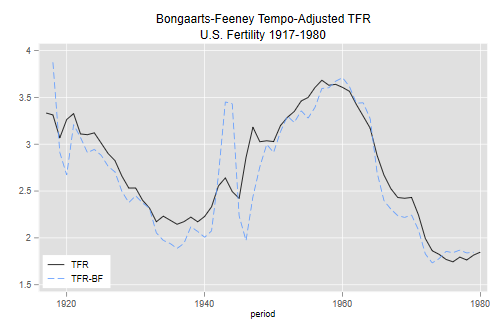```> periods <- mutate(periods,
+   rp = nder(mac),
+   tfrs = tfr/(1 - rp))
> ggplot(periods,aes(year,tfr)) + geom_line() +
+   geom_line(aes(year,tfrs), linetype=2)  +